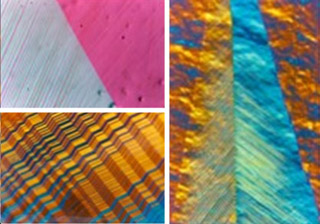Course Description
This course forms an introduction to a selection of mathematical topics that are not covered in traditional mechanical engineering curricula, such as differential geometry, integral geometry, discrete computational geometry, graph theory, optimization techniques, calculus of variations and linear algebra. The topics …
Course Info
Instructor
Departments
Topics
Learning Resource Types